/

### Estimating operational risk capital requirements assuming data follows a bi-exponential distribution

Suppose a risk manager believes that an appropriate model for a particular type of operational risk exposure involves the loss,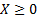, coming 50% of the time from an exponential distribution with parameter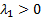and 50% of the time come from an exponential distribution with parameter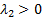. The exponential distribution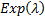has a probability density function,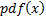, mean,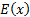, and variance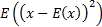as follows: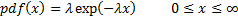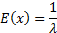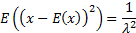Suppose we want method of moments (MoM) estimators for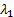and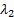and we have loss data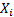for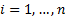where the number of losses,, is sufficiently large to be able to ignore small sample corrections. Then the MoM estimators can be derived as follows, where the (sample) moments used in the estimation are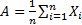and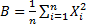.

The pdfs of the individual parts,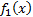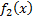, and of the overall distribution,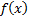, are: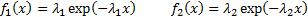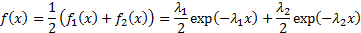The mean ofis: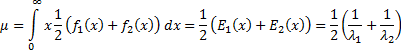where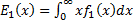and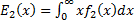By integrating by parts or by noting that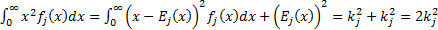for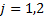where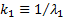and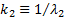, we note that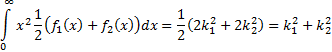So method of moments estimators involve (if these simultaneous equations have a (real) solution):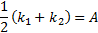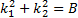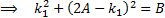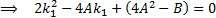This is a quadratic equation which has the following solutions: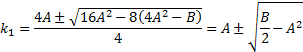The two roots correspond to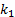and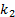(it is not possible to differentiate between them given merely the data being provided). Hence the values ofandare: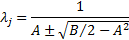In practice it is more likely that the probabilities of drawing from the underlying exponentials are unknown. This adds an extra degree of freedom which would introduce the need to include a further (higher) moment into the parameter estimation process.